# Introduction to Forward Propagation in Neural Networks

A Neural Network is a DAG (Directed Acyclic Graph) where the data samples as input provided to the Input layer flow in the defined forward direction. As the movement happens in the forward direction, we call the process Forward Propagation. Because of this property, ANNs are also called "feed-forward" networks. This is an important concept in ANN theory. In this article, we focus on discussing it in detail by actually performing forward propagation through a dummy ANN architecture.

#### Key takeaways from this blog

After going through this blog, we will be able to understand the following things:

1. What are the various working steps in a Neural Network?
2. What is forward propagation in Neural Networks?
3. What is a feed-forward network?
4. Implementation of Forward propagation.

#### Quick-Revision on Working Steps For a Neural Network

If we recall the methodology through which a machine learning model works, we can recall these steps:

Step 1: We first finalize the input features to pass them through the input layer. The number of nodes in the Input layer will be equal to the number of features present in our data.

Step 2: This input is passed to the hidden layer/s (if present) in the neural networks, where important information is extracted from data that can help in learning. During this extraction process, input features get transformed after several rounds of matrix multiplication and passing through non-linear activation functions. We can divide the processes happening in hidden layers into two steps:

• Preactivation: Here, the linear transformation happens for the input received. For the first hidden layer, input is received from the Input layer, and for the latter hidden layers, input is received from the previous hidden layer. A linear transformation is the weighted sum of the input and bias values. In simple mathematical form, we represent this transformation as W.T * X + B (Transposed weight multiplied by input, and the result is added with a bias value).
• Activation: The calculated value from the preactivation part is then sent to the activation function, where non-linear transformation happens. For every layer, we define the activation function, and the transformation varies based on the nature of the selected function. For example, in the case of the ReLU activation function, any negative values formed from the linear transformation step in preactivation will be forced to zero.

Step 3: This transformed output of hidden layers is passed to the output layer, where it gets transformed further to convert it into the desired format. The desired format can be a single integer, a floating number, or even a vector/matrix of numbers.

Step 4: There can be two ML model development scenarios: training or testing a Neural Network.

• Training: When training a Neural Network, the output from the Output layer is compared with the true labels in the data. This comparison is used to calculate the cost function used to train ANNs. Optimizers update the weight and bias values and help in finding the minimum cost value. To update these parameters, we send the cost values back into the network (Output to Input). This process of sending the data back and updating the previously used weight values is known as backpropagation, and we will learn about this in a separate blog.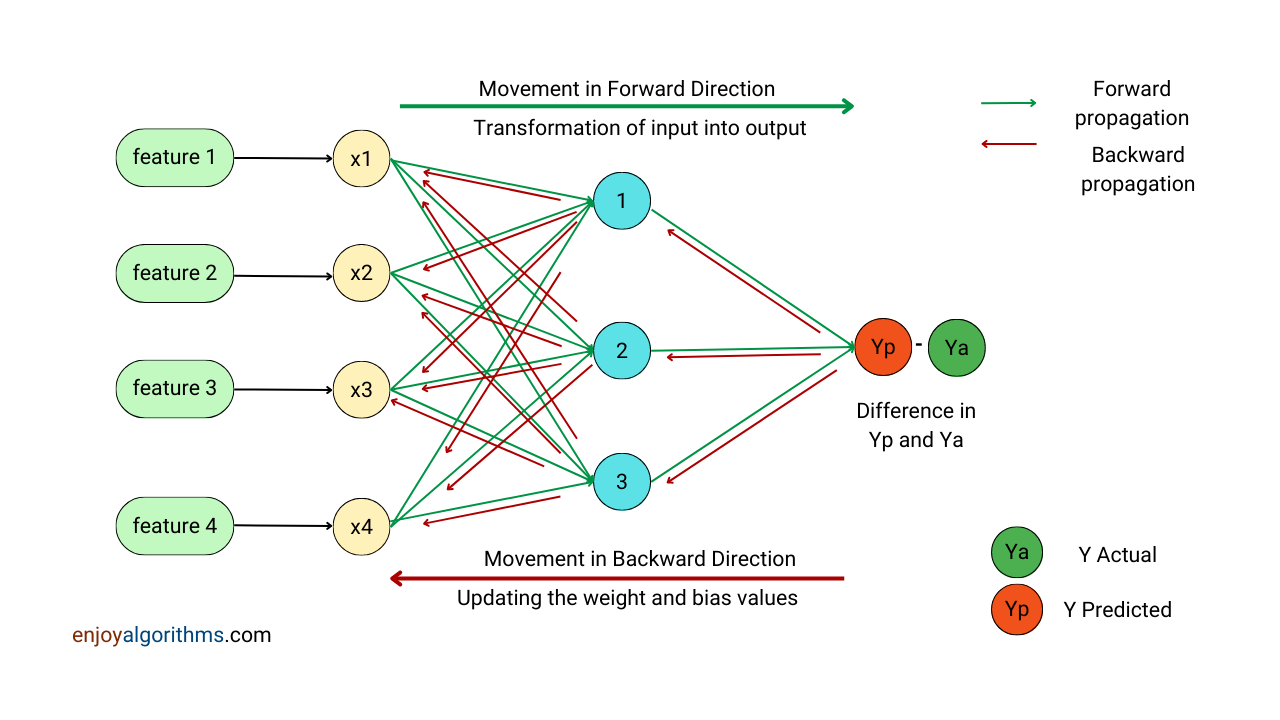• Testing: In the case of testing (or inferencing) an already trained Neural Network, the output produced by the Output layer is treated as the final predicted output by the model. At this time, data only moves in a forward direction. Because of this property, an ANN is also a feed-forward network, and this entire process of inferencing using input features is forward propagation.

#### What is forward propagation in Neural Networks?

If we break down the name, forward means the forward way, and propagation means spreading out. So, in combination, forward propagation means moving only in the forward direction.

In a neural network, the journey of input features being transformed into the output after passing through one/multiple hidden layers and the output layer is known as forward propagation.

A Neural Network is a Directed Acyclic Graph (DAG) where we define the direction from one node to the other. This direction is always defined in the forward direction (Input to Ouptut), and data samples follow this direction to get transformed into the desired output value/s. This movement of propagating the forward direction is known as forward propagation. To understand the fundamentals clearly, let's see the mathematics and the implementation of forward propagation in greater detail.

### Mathematical Implementation of Forward Propagation

We will need dummy data and a dummy NN architecture to propagate forward.

#### Preparation of Dataset

We will create a dummy dataset using the make_blobs function from the Scikit-learn library. It generates isotropic Gaussian blobs for clustering. As the Neural Networks is a supervised learning algorithm, let's generate a 'two class' labeled dataset to keep the flow simple to understand.

``````from sklearn.datasets import make_blobs
from matplotlib import pyplot as plt

X, y = make_blobs(n_samples=100, centers=2, n_features=2, random_state=0)

plt.figure()
plt.scatter(X[:, 0], X[:, 1], c = y, s = 50, cmap = 'RdBu')
plt.show()``````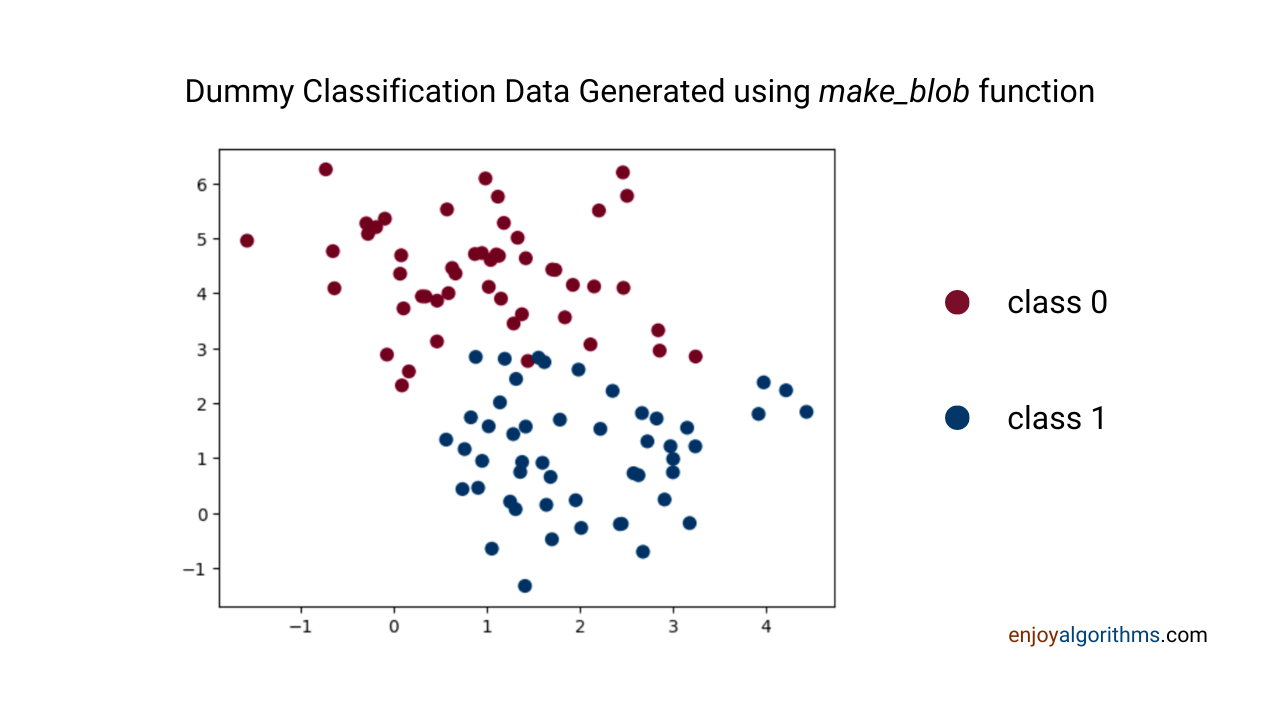#### Preparation of Neural Network Architecture

We will use a Neural Network with a single hidden layer and 2 nodes to easily understand the flow. Also, the choice for activation function will be sigmoid in both hidden and output layers. If we use sigmoid as the activation function in the output layer for a binary classification problem, the number of nodes in the output layer will be 1.

The schematic diagram and corresponding notations are given in the diagram below.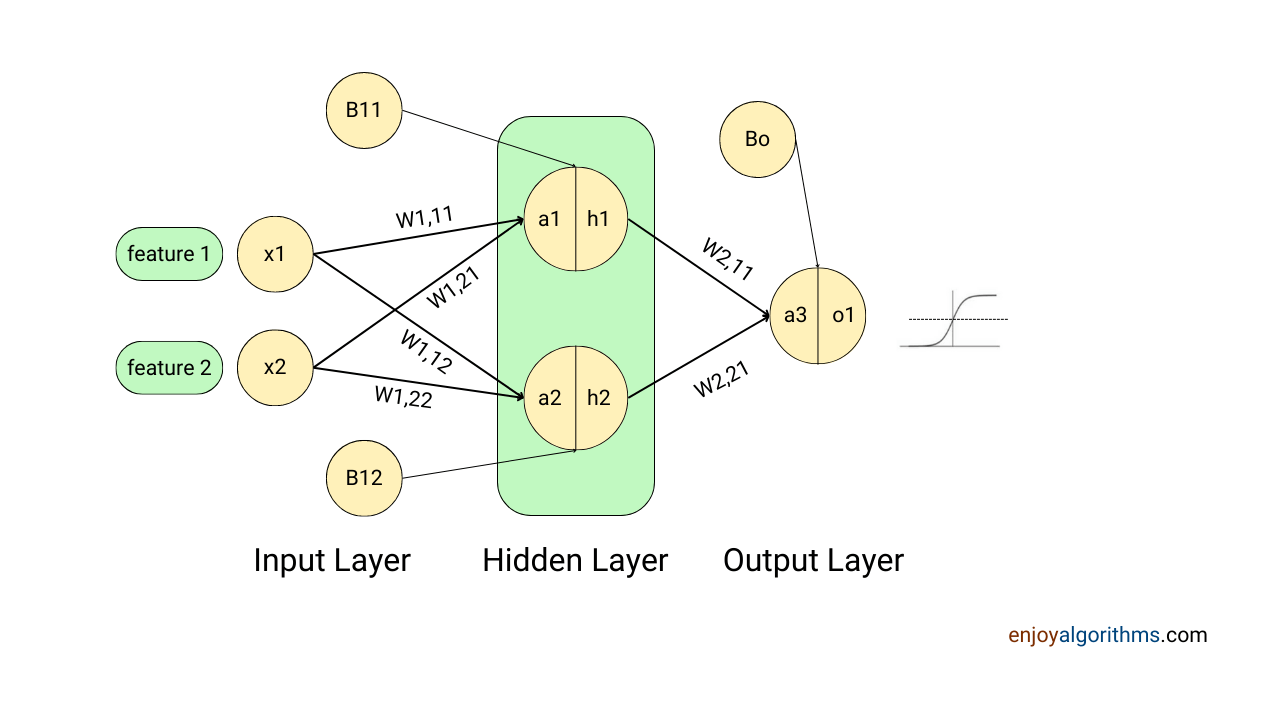In our components of the Artificial Neural Network blog, we mentioned that there would be one weight value for every connection in a neural network. Also, there will be one bias value for every node in the hidden and output layer. The above diagram represents six weights for six connections and three biases for three nodes (2 nodes in the hidden layer and 1 node in the output layer).

While training an ANN, data passes through multiple forward and backward propagation. For the first pass of forward propagation, we need to provide some starting points for these weight and bias values, also called weight initialization. In most cases, we initialize these values randomly.

During training, through multiple rounds of forward and backwardpropagation, machines find the perfect values for these weight and bias values to train the model successfully. This perfectness is nothing but finding those values for which the average difference (or squared distance) between the true and the predicted values becomes minimum. In every backpropagation pass, machines update the weight and bias values to achieve the perfect values.

### Weight Initialization in Neural Networks

There are two ways of initializing the parameters:

1.  Initializing every six weights and three biases separately,
2. Define the matrix for weight values and perform matrix multiplication.

Let's see both ways.

#### Method 1: Initializing every value separately:

We can use the numpy library to assign the random values to all the parameters involved in the learning process.

``````import numpy as numpy

## Random initialization happened for six weights
w111 = np.random.randn()
w112 = np.random.randn()
w121 = np.random.randn()
w122 = np.random.randn()
w211 = np.random.randn()
w212 = np.random.randn()

## Random initialization happened for three biases
b11 = np.random.randn()
b12 = np.random.randn()
bo = np.random.randn()``````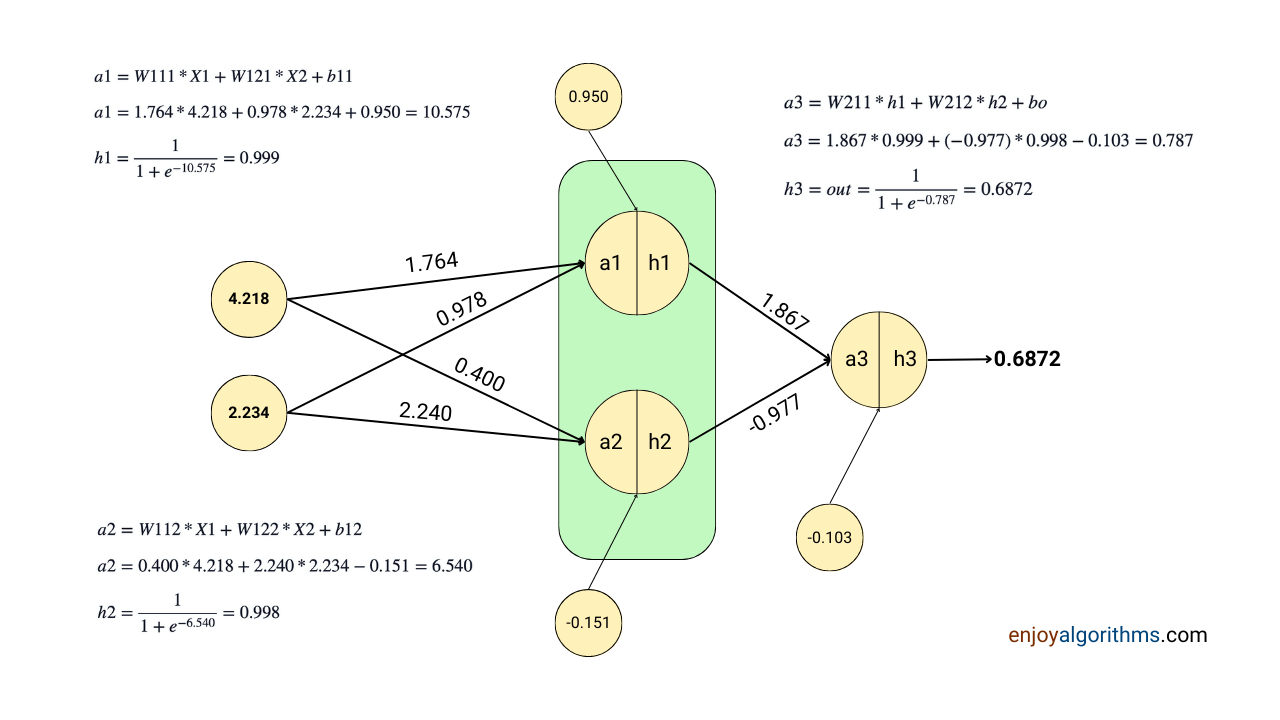Forward Propagation Implementation in Python from Scratch:

``````from sklearn.datasets import make_blobs
import numpy as np

X, y = make_blobs(n_samples=1000, centers=2, n_features=2, random_state=0)

## Defining the sigmoid activation function
def sigmoid(x):
temp = 1/(1 + np.exp(-x))
return temp

## Defining the forward propagation function

def forward_pass(x): # Here X will have both components X1, and X2

## Inputs X1 and X2
X1, X2 = X

## Node 1 of first hidden layer
a1 = X1*w111 + X2*w121 + b11
h1 = sigmoid(a1)

## Node 2 of first hidden layer
a2 = X1*w112 + X2*w122 + b12
h2 = sigmoid(a2)

## The output node
a3 = h1*w211 + h2*w212 + bo
out = sigmoid(a3)

return out

out = forward_pass(X)

out_prob = out*100

## 67.75 %``````

The output generated here can be treated as the probability value as the last node uses the sigmoid activation function. We can easily set the threshold value for the output of the sigmoid function. For example, if the output probability is greater than 60%, predict class 1. And if the output probability is less than 60%, predict class 0. If you want to explore the sigmoid activation function, please read this blog for a detailed discussion.

Initializing every parameter separately and then performing the calculation could be more computationally efficient. In some ANN applications, these parameters can range in millions, and we won't be able to initialize them separately. Hence, we use matrix multiplication for this. Let's see.

#### Method 2: Using Matrix Multiplication for Forward Propagation

In the design architecture of ANN, there are two input variables, X1 and X2. If we pass it as a vector, the shape will be (2 x 1). We have two nodes in the hidden layer and 1 node in the output layer. Hence, the weight matrix used between the hidden and input layers will be of shape (2 x 2), and the weight matrix between the hidden and output layers will be of shape (2 x 1).

But how did we achieve these matrix dimensions? To understand this, let's dive into the mathematical details. We might know that the mathematical equation for linear transformation happening during preactivation is:

``pre-activation = W.T*X + B ## Weight transpose * Input + Bias``

We already know that the input matrix X has a shape (2 x 1), which will be multiplied by the transpose of the weight matrix (W.T). Hence, the weight matrix should have the first dimension equal to the first dimension of the input, which is 2. But why the first dimension only?

Because we are not directly multiplying the Weight matrix with the Input matrix X, we are first transposing it. So, to multiply with a (2 x 1) matrix, the 'weight transpose' should have the last dimension of 2. Hence, weight should have a first dimension of 2.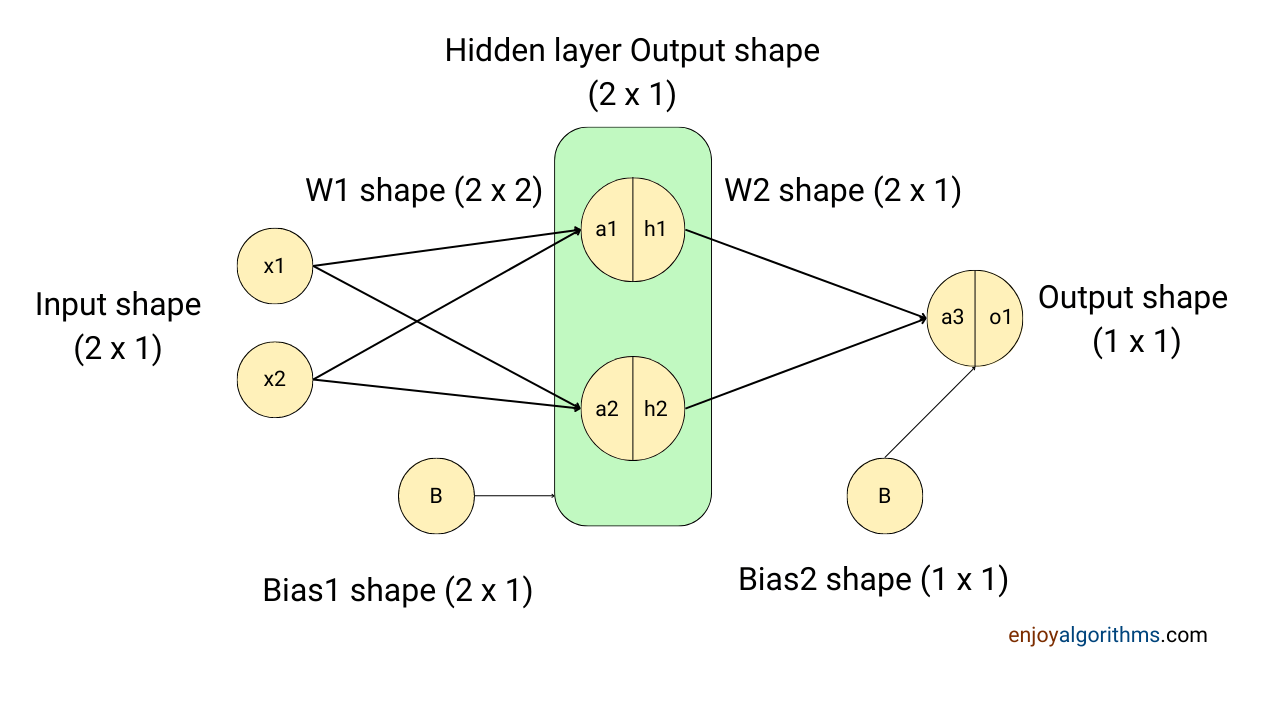Now, the hidden layer has two nodes, which will be treated as the input for later layers. So, the second dimension of the weight matrix will also be 2, making the final dimension of the weight matrix between the hidden and input layers (2 x 2). The resultant matrix with the multiplication of W.T and X would be (2 x 1), and to make the matrix addition valid, B will also have the shape of (2 x 1).

Similarly, we know the output layer has 1 node, and the final output we expect is a shape of (1 x 1). The output of the hidden layer will have a shape of (2 x 1). Hence, the weight matrix between the hidden and output layers will have a shape (2 x 1) so that the transpose will be (1 x 2), and the matrix multiplication will produce (1 x 1) output. Again, to make the matrix addition valid, the bias matrix for the Output layer will have a shape (1 x 1).

Implementation in Python

``````from sklearn.datasets import make_blobs
import numpy as np

X, y = make_blobs(n_samples=1000, centers=2, n_features=2, random_state=0)

W1 = np.random.randn(2,2)
W2 = np.random.randn(2,1)
B1 = np.zeros((2,1))
B2 = np.zeros((1,1))

def forward_pass_matmul(x): # Here X will have both components X1, and X2

a_hidden = np.matmul(W1.T, x) + B1
h_hidden = sigmoid(a_hidden)

a_out = np.matmul(W2.T, h_hidden) + B2
h_out = sigmoid(a_out)

return h_out``````

This code seems much cleaner now and more efficient than the earlier implementation. With the same hypothesis used in Method 1, we can decide which class to predict by setting a threshold.

This is it for this blog. In our next blog, we will learn about another important concept of backward propagation for ANNs in greater detail.

### Conclusion

The movement of data samples in the forward direction of an Artificial Neural Network is known as Forward Propagation. At the time of inference from an already trained model, we perform only forward propagation, and for this, ANNs are also called feed-forward networks. This article discussed the basics of forward propagation by implementing it on dummy data and ANN architecture. We hope you enjoyed the article and become ready to understand backpropagation.

Enjoy Learning!

☆ 16-Week Live DSA Course
☆ 10-Week Live DSA Course